Latest Banking jobs   »

# Quantitative Aptitude Quiz For FCI Phase I 2023- 10th January

Direction (1 – 5): In the following questions, there are two equations in x and y. You have to solve both the equations and give answer.

Q1. I. 2x²+ 15x + 28 = 0
II. y² + 6y + 8 = 0
(a) if x > y
(b) if x < y
(c) if x ≥ y
(d) if x ≤ y
(e) if x = y or there is no relation between x and y

Q2. I. x = ∛343
II. y² – 13y+42= 0
(a) if x ≤ y
(b) if x < y
(c) if x ≥ y
(d) if x > y
(e) if x = y or there is no relation between x and y

Q3. I. 4x² + 11x + 6 =0
II. y² + 5y + 6 = 0
(a) if x ≤ y
(b) if x > y
(c) if x = y or there is no relation between x and y
(d) if x < y
(e) if x ≥ y

Q4. I. 6x² –x – 1 = 0
II. 8y² – 2y – 1 = 0
(a) if x ≤ y
(b) if x > y
(c) if x = y or there is no relation between x and y
(d) if x < y
(e) if x ≥ y

Q5. I. x²-7x+12=0
II. y²-2y-3=0
(a) if x ≤ y
(b) if x ≥ y
(c) if x = y or there is no relation between x and y
(d) if x < y
(e) if x > y

Directions (6–10): In the following questions, there are two equations in x and y. You have to solve both the equations and give answer.

Q6. I. x²-7x+12=0
II. y²+4y-5=0
(a) if x > y
(b) if x < y
(c) if x ≥ y
(d) if x ≤ y
(e) if x = y or there is no relation between x and y

Q7. I. x²-29x-30=0
II. y²-3y-10=0
(a) if x > y
(b) if x < y
(c) if x ≥ y
(d) if x ≤ y
(e) if x = y or there is no relation between x and y

Q8. I. x²-10x+25=0
II. y²-25y+150=0
(a) if x > y
(b) if x < y
(c) if x ≥ y
(d) if x ≤ y
(e) if x = y or there is no relation between x and y

Q9. I. x²-2x-15=0
II. y²-4y-5=0
(a) if x > y
(b) if x < y
(c) if x ≥ y
(d) if x ≤ y
(e) if x = y or there is no relation between x and y

Q10. I. x²+8x+16=0
II. y²+12y+11=0
(a) if x > y
(b) if x < y
(c) if x ≥ y
(d) if x ≤ y
(e) if x = y or there is no relation between x and y

Direction (11-15): In each of these questions, two equation (I) and (II) are given. You have to solve both the equations and give answer.
(a) If x>y
(b) If x≥y
(c) If x<y
(d) If x≤y
(e) If x = y or no relation can be established between x and y

Q11. I. 16x²+44x+24=0
II. y³=8

Q12. I. x²-8x+15=0
II. y²-5y+6=0

Q13. I. x²+4x-96=0
II. y²-2y-3=0

Q14. I. x²-7x+12=0
II.y²+3y+2=0

Q15. I. x²-13x+42=0
II. y²-16y+63=0

Solutions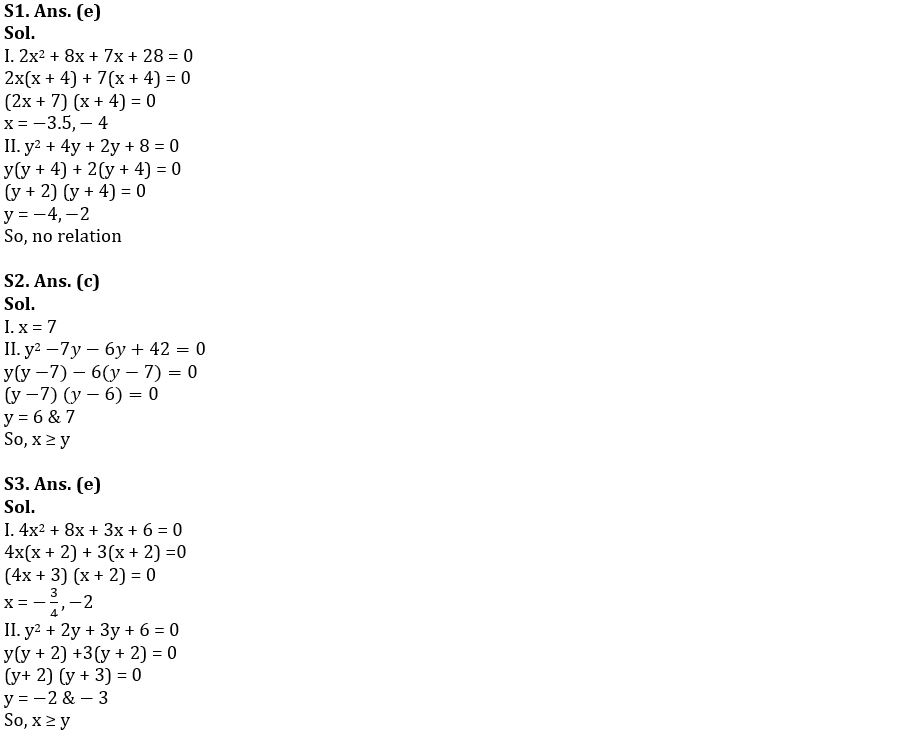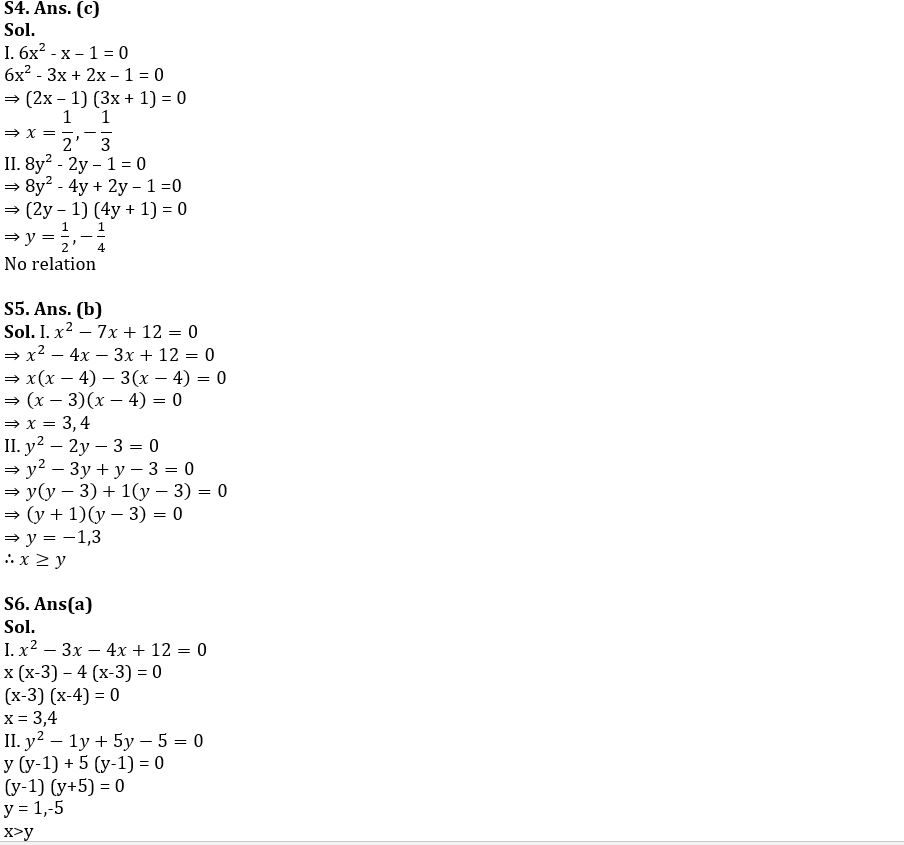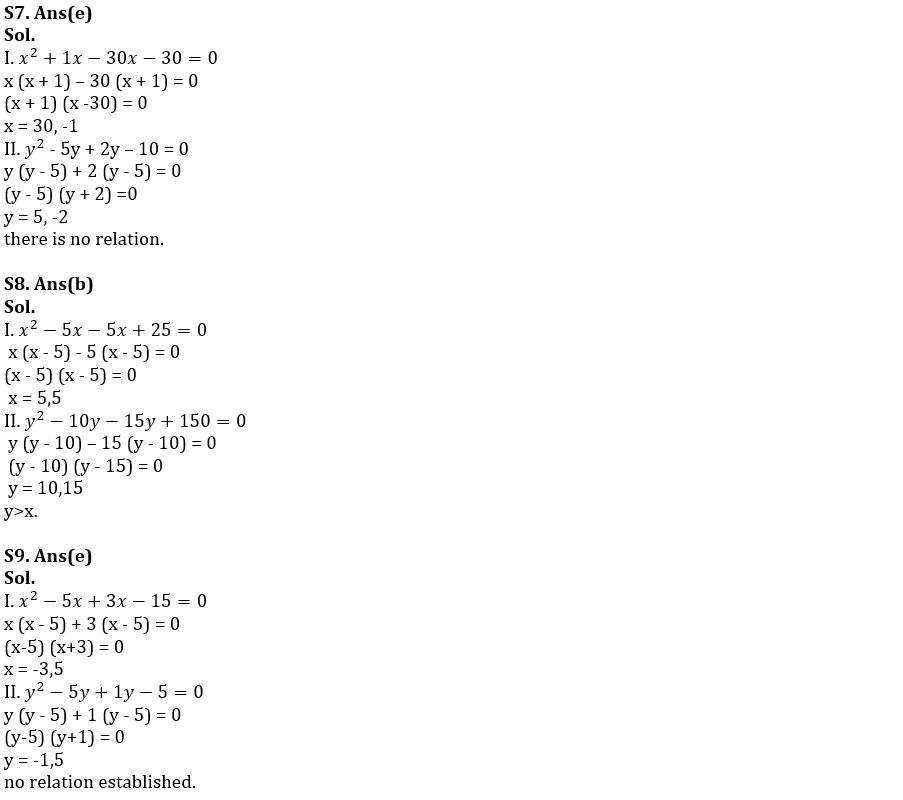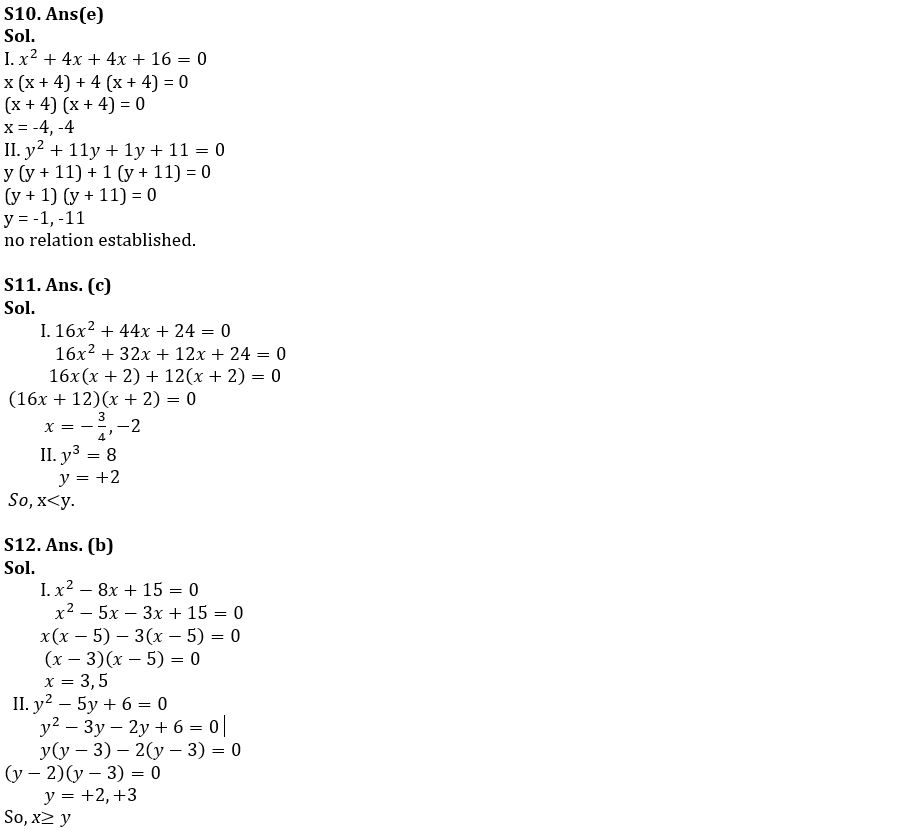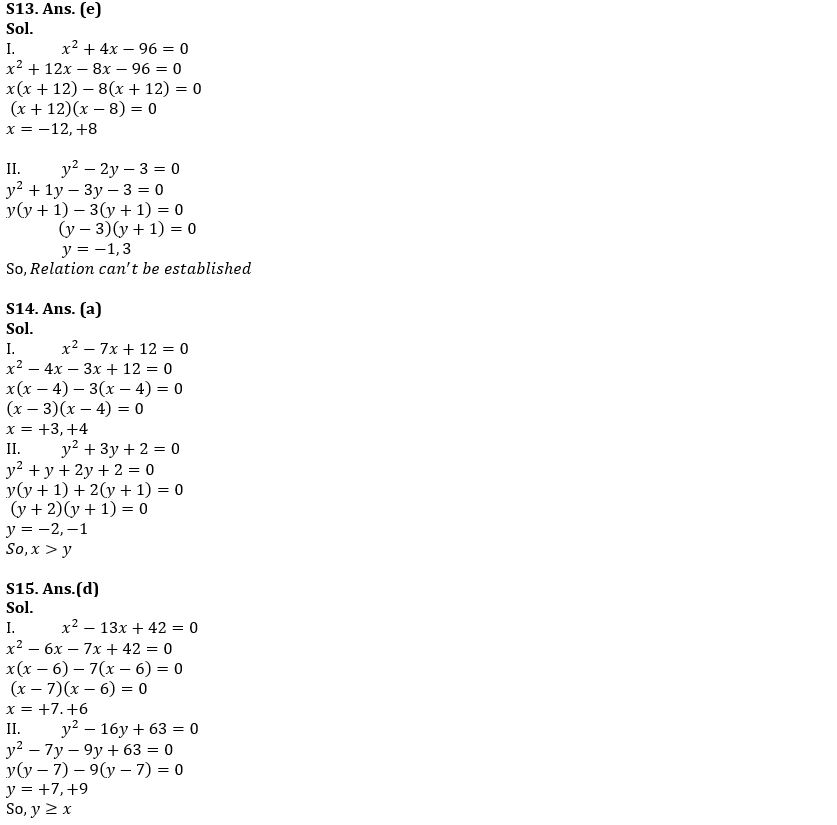## FAQs

### What is the FCI Assistant Grade 3 Phase 1 Exam Date 2023?

The FCI Assistant Grade 3 Phase 1 Exam Dates 2023 are 1st, 7th, 14th & 21st January 2023.

#### Congratulations!Union Budget 2023-24: Free PDF